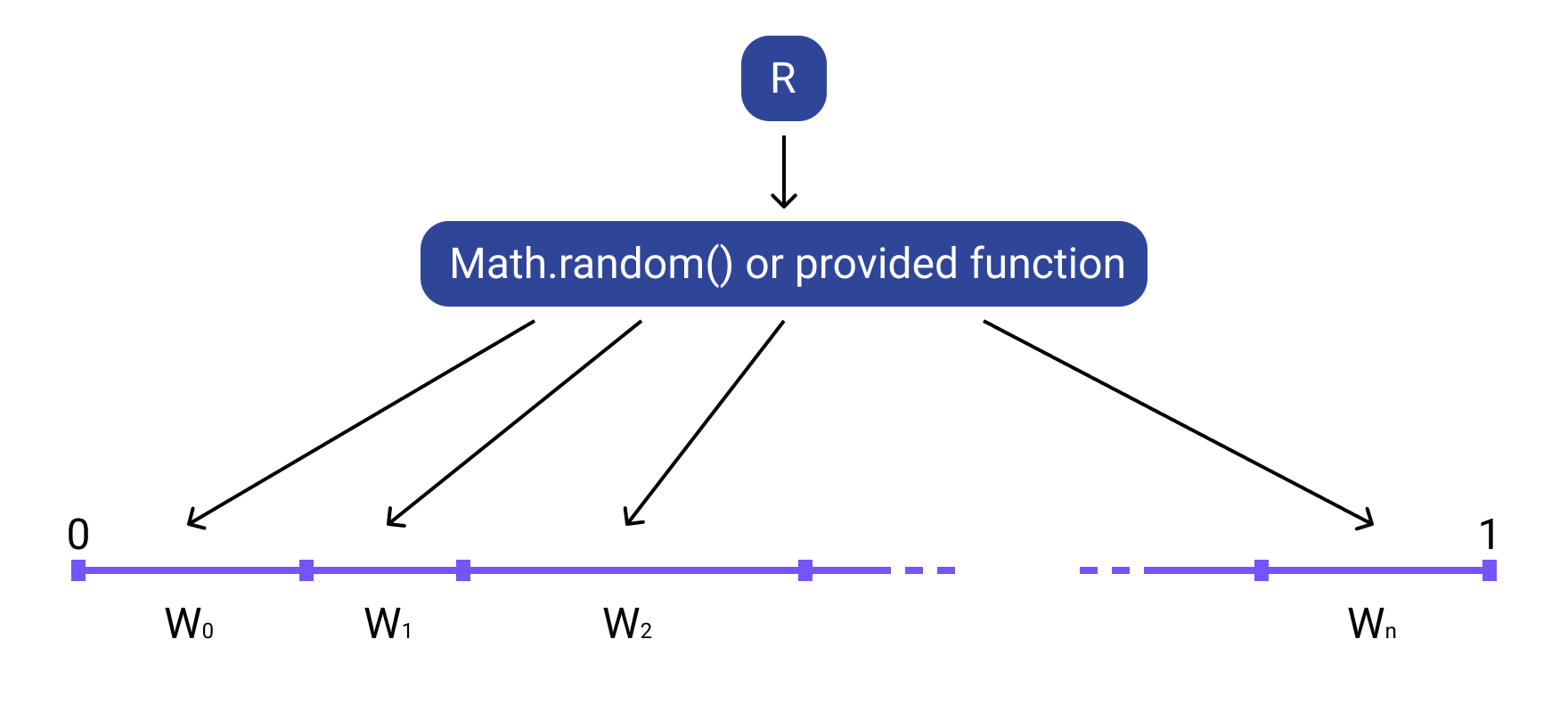Library with helper methods to work with weighted random.

## Usage no npm install needed!

``````<script type="module">
</script>``````

Tiny library that provides simple API for weighted random. It works like that:## Start

Install dependency:

`npm install @sharadbrat/randomizer`

Import in project:

``````import Randomizer from '@sharadbrat/randomizer';
``````

Use:

``````const r = new Randomizer([
[1, 'A'],
[1, 'B'],
[1, 'C'],
]);

r.choose(); // will be 'A', 'B', or 'C' with equal probability
``````

## Examples

Weights define the probability, according to this formula: `{weight} / {sum_of_weights}`

``````const r = new Randomizer([
[1, 'A'],
[3, 'B'],
]);

r.choose(); // will be 'A' with 0.25 probability or 'B' with 0.75 probability
``````

## Testing

`npm run test`

Runs tests with mocha.